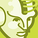# App Development

Announcements
April 22, 2PM EST: Learn about GeoOperations in Qlik Sense SaaS READ MORE
cancel
Showing results for
Did you mean:Creator

## \$ expansion of a conditional function in load editor, load as a field

Hello I have this variable

Set f_GroupScore =
'If( (Sum(ACDTIME) + Sum(ACWTIME) + Sum(HOLDTIME)) / Sum(ACDCALLS) >= GroupScore1,
1,
If( (Sum(ACDTIME) + Sum(ACWTIME) + Sum(HOLDTIME)) / Sum(ACDCALLS) >= GroupScore2,
2,
If( (Sum(ACDTIME) + Sum(ACWTIME) + Sum(HOLDTIME)) / Sum(ACDCALLS) >= GroupScore3,
3,
If( (Sum(ACDTIME) + Sum(ACWTIME) + Sum(HOLDTIME)) / Sum(ACDCALLS) >= GroupScore4,
4,
If( (Sum(ACDTIME) + Sum(ACWTIME) + Sum(HOLDTIME)) / Sum(ACDCALLS) >= GroupScore5,
5,
If( (Sum(ACDTIME) + Sum(ACWTIME) + Sum(HOLDTIME)) / Sum(ACDCALLS) >= GroupScore0,
1,
Null()
)
)
)
)
)
)';

I am trying to load it as a field but in the table it isn't expanding.  I loaded it as:

AHT:
KEY_IdDate,
REPORT_GROUP,
GroupScore1,
GroupScore2,
GroupScore3,
GroupScore4,
GroupScore5,
GroupScore0,
ACDCALLS,
ACDTIME,
HOLDTIME,
ACWTIME,
TOTALACDCALLS,
ACDCALLS/TOTALACDCALLS as Weight,
'\$(f_GroupScore)'*(ACDCALLS/TOTALACDCALLS) as Weight1
resident Temp2_AHT
;

I want to expand it in the load editor so I can then filter certain values from showing up in the grids but instead of evaluating the expression it is only returning the string of the expression.  I've tried 'set', and 'let', I've tried inputing '=' in the parantheses.  Not sure what I'm missing is it not evaluating because it is an if statement?  Any help would be appreciated.

Thanks,

1 Solution

Accepted SolutionsMVP & Luminary

The variable is neither properly created nor called - try it with (here simplified for the syntax):

set exp = "sum(Field)";

t: load F1, F2, \$(exp) as F3 from Source group by F1, F2;

By your intended aggregating you will further need an appropriate group by to all fields which aren't included in the aggregations. This means you may not able to apply the aggregating within your origin load because the other fields may prevent the aggregation on the intended level - in this case you need to do the aggregation on the outside and map or join the results together.

Beside this you use the + operator - if any of the parameter didn't return a valid numeric value the result will be NULL. If this is possible but not intended you should use rangesum() because each non numeric result will be treated as 0.

- Marcus

2 RepliesMVP & Luminary

The variable is neither properly created nor called - try it with (here simplified for the syntax):

set exp = "sum(Field)";

t: load F1, F2, \$(exp) as F3 from Source group by F1, F2;

By your intended aggregating you will further need an appropriate group by to all fields which aren't included in the aggregations. This means you may not able to apply the aggregating within your origin load because the other fields may prevent the aggregation on the intended level - in this case you need to do the aggregation on the outside and map or join the results together.

Beside this you use the + operator - if any of the parameter didn't return a valid numeric value the result will be NULL. If this is possible but not intended you should use rangesum() because each non numeric result will be treated as 0.

- MarcusCreator
Author

Hi Marcus,

That worked. I changed the null() in my expression to a 0 which works for what I'm intending, and in the subsequent load statement I did the group by like you suggested included all the attributes to group by.  I was able to load the variable now as a field and it worked and shows up evaluated in the straight table in my visualizations like I wanted.

Thanks!Tags
Community Browser